# Selina Solutions Concise Mathematics Class 6 Chapter 25 Properties of Angles and Lines Exercise 25(B)

Selina Solutions Concise Mathematics Class 6 Chapter 25 Properties of Angles and Lines Exercise 25(B) contains descriptive answers, in accordance with the latest ICSE syllabus. An angle formed by two lines and their transversal lines is the main concept talked about under this exercise. The main objective of creating solutions by the experts in a lucid manner, is to impart better understanding among students. For further details, students can avail Selina Solutions Concise Mathematics Class 6 Chapter 25 Properties of Angles and Lines Exercise 25(B) PDF, from the links mentioned below for free download.

## Selina Solutions Concise Mathematics Class 6 Chapter 25 Properties of Angles and Lines Exercise 25(B) Download PDF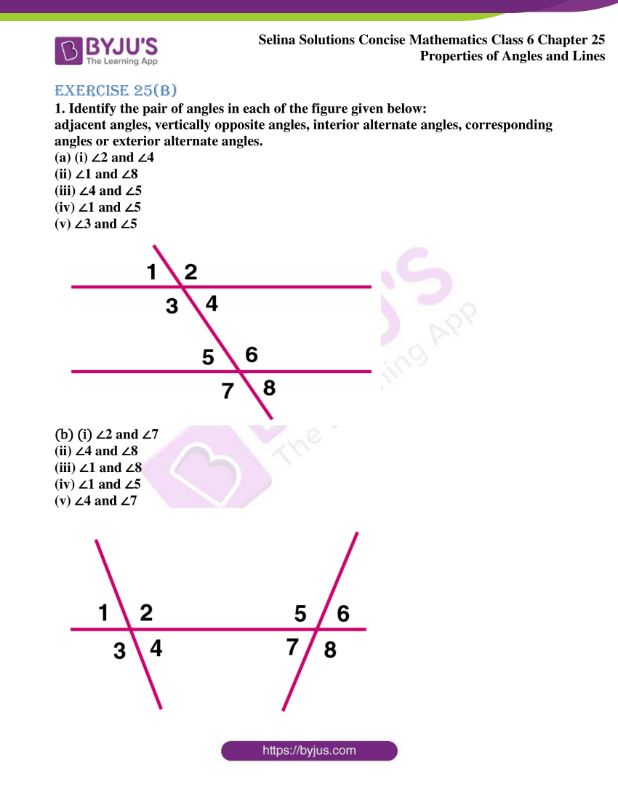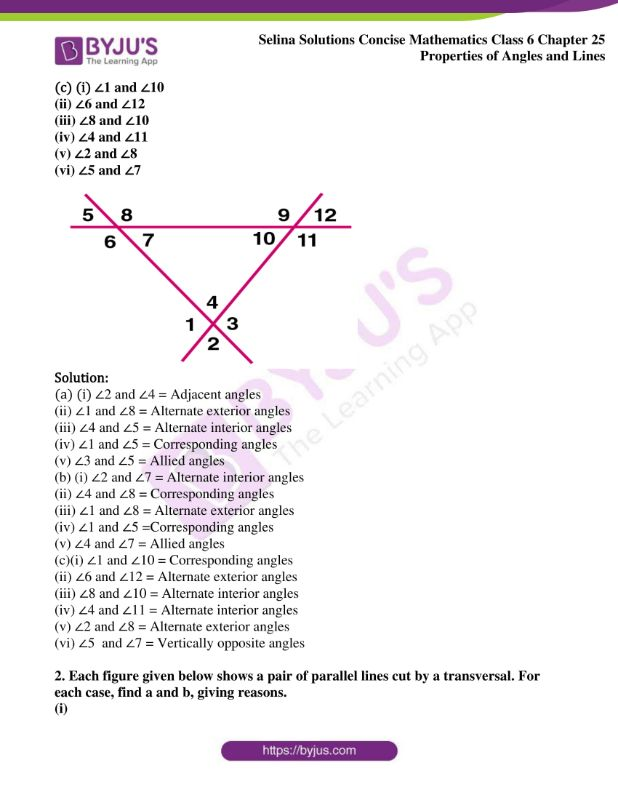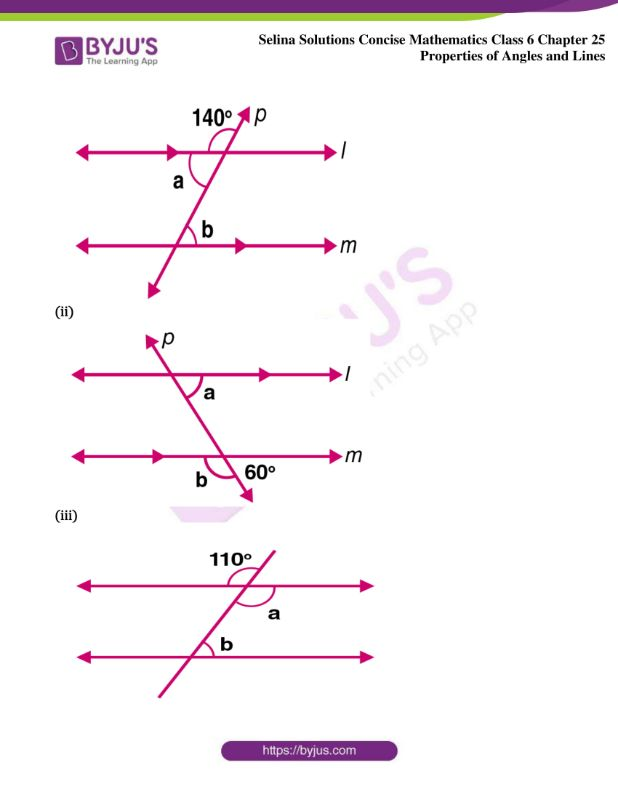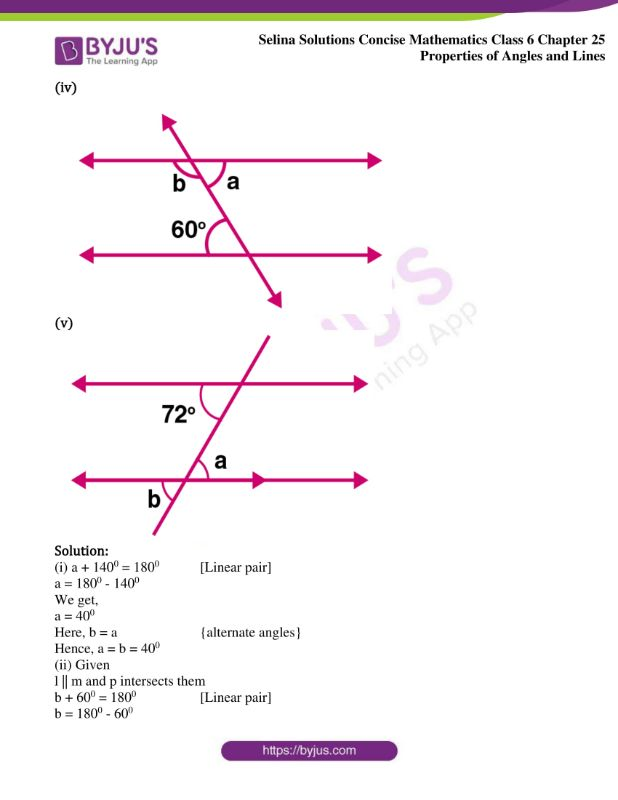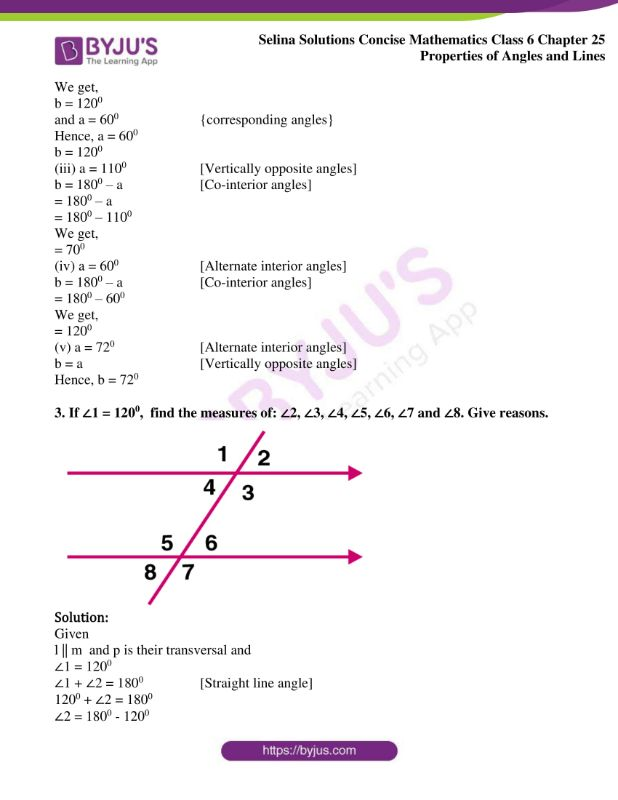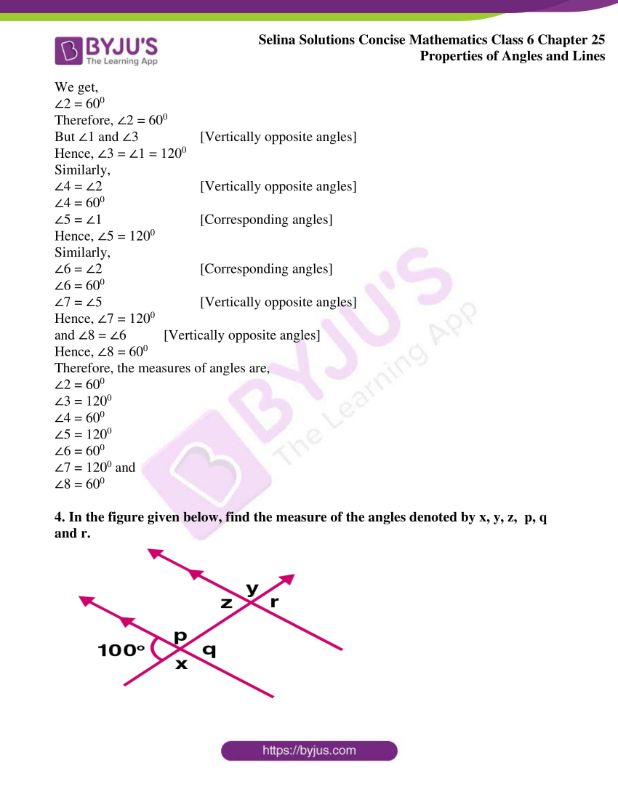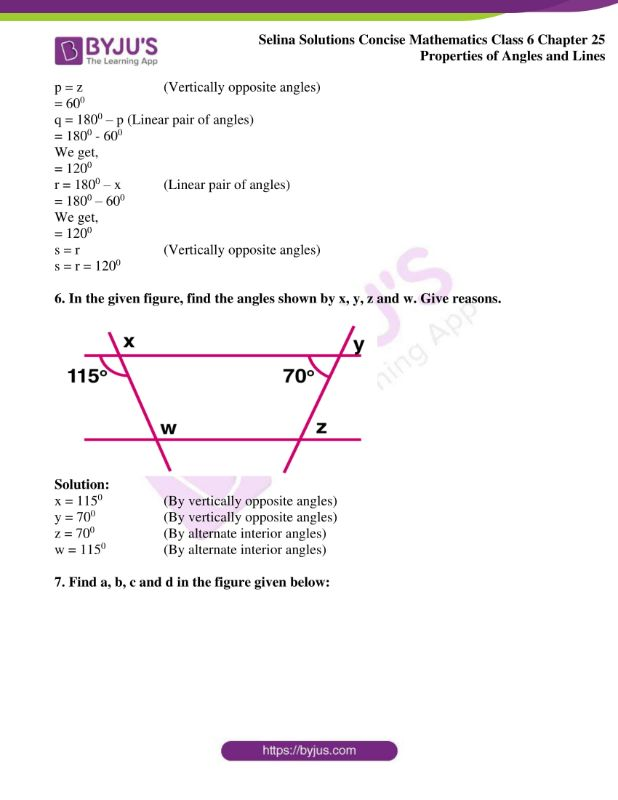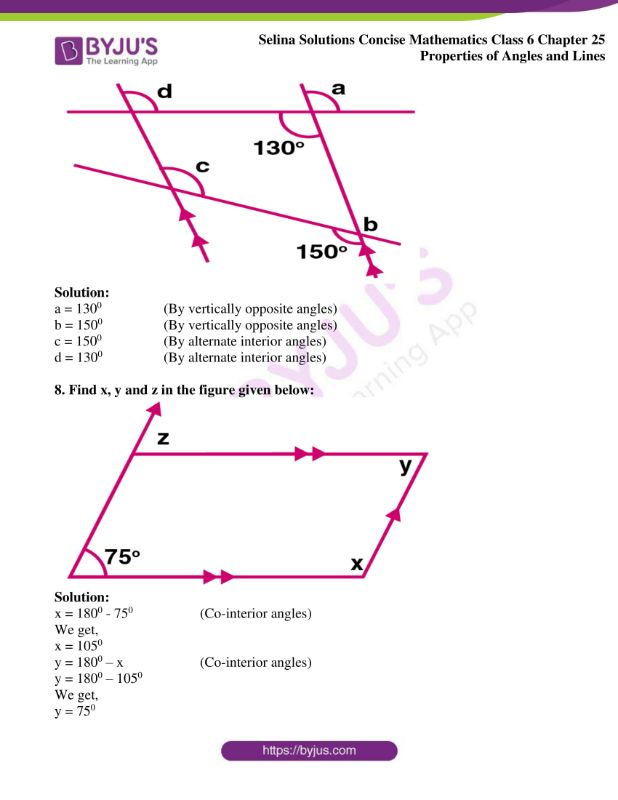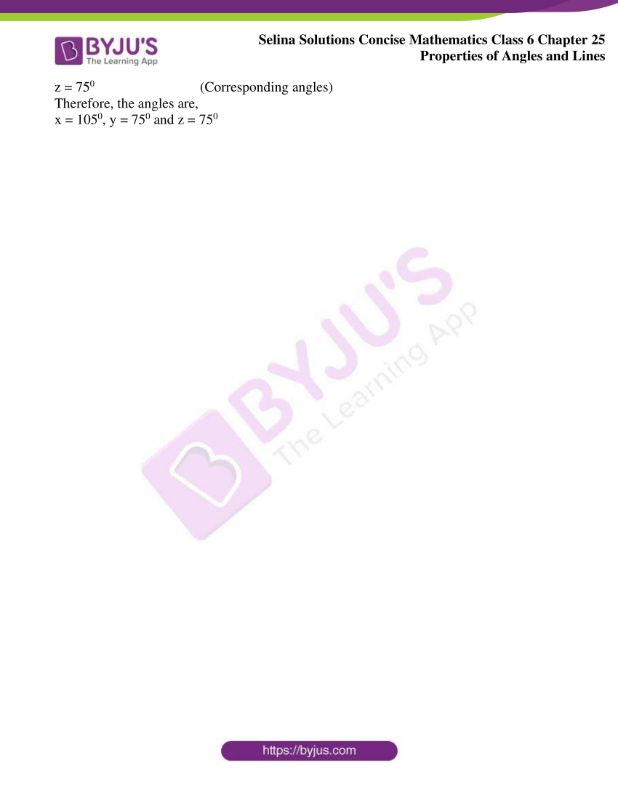### Access Selina Solutions Concise Mathematics Class 6 Chapter 25 Properties of Angles and Lines Exercise 25(B)

Exercise 25(B)

1. Identify the pair of angles in each of the figure given below:

adjacent angles, vertically opposite angles, interior alternate angles, corresponding angles or exterior alternate angles.

(a) (i) ∠2 and ∠4

(ii) ∠1 and ∠8

(iii) ∠4 and ∠5

(iv) ∠1 and ∠5

(v) ∠3 and ∠5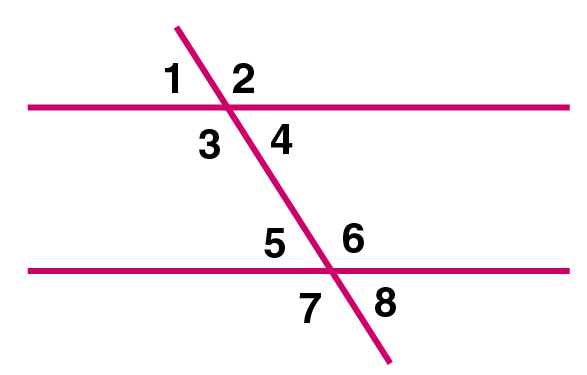(b) (i) ∠2 and ∠7

(ii) ∠4 and ∠8

(iii) ∠1 and ∠8

(iv) ∠1 and ∠5

(v) ∠4 and ∠7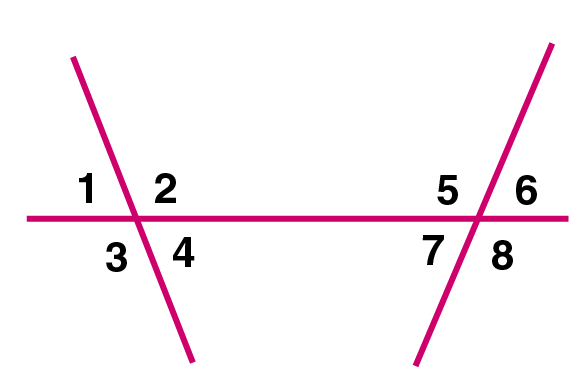(c) (i) ∠1 and ∠10

(ii) ∠6 and ∠12

(iii) ∠8 and ∠10

(iv) ∠4 and ∠11

(v) ∠2 and ∠8

(vi) ∠5 and ∠7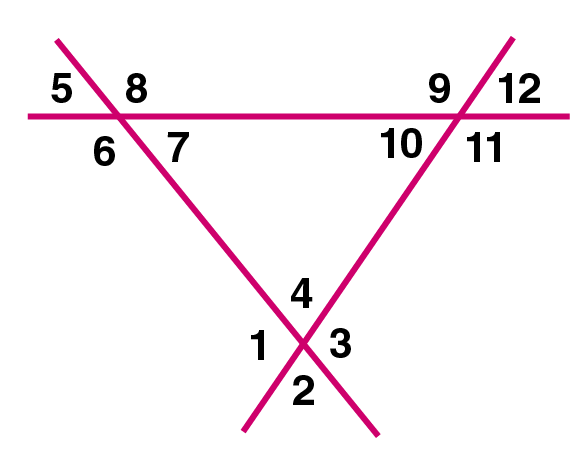Solution:

(a) (i) ∠2 and ∠4 = Adjacent angles

(ii) ∠1 and ∠8 = Alternate exterior angles

(iii) ∠4 and ∠5 = Alternate interior angles

(iv) ∠1 and ∠5 = Corresponding angles

(v) ∠3 and ∠5 = Allied angles

(b) (i) ∠2 and ∠7 = Alternate interior angles

(ii) ∠4 and ∠8 = Corresponding angles

(iii) ∠1 and ∠8 = Alternate exterior angles

(iv) ∠1 and ∠5 =Corresponding angles

(v) ∠4 and ∠7 = Allied angles

(c)(i) ∠1 and ∠10 = Corresponding angles

(ii) ∠6 and ∠12 = Alternate exterior angles

(iii) ∠8 and ∠10 = Alternate interior angles

(iv) ∠4 and ∠11 = Alternate interior angles

(v) ∠2 and ∠8 = Alternate exterior angles

(vi) ∠5 and ∠7 = Vertically opposite angles

2. Each figure given below shows a pair of parallel lines cut by a transversal. For each case, find a and b, giving reasons.

(i)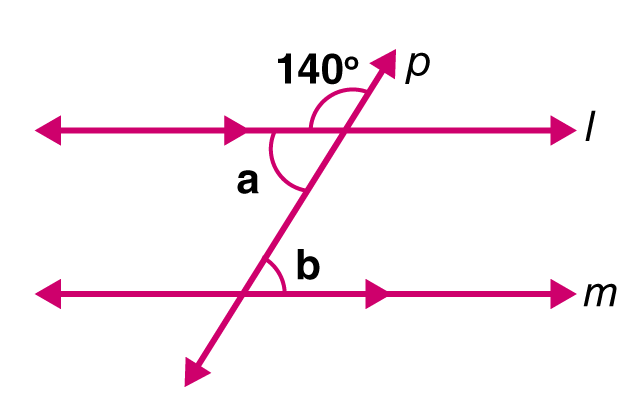(ii)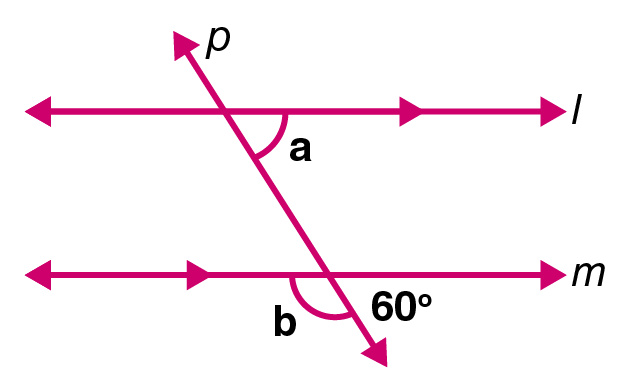(iii)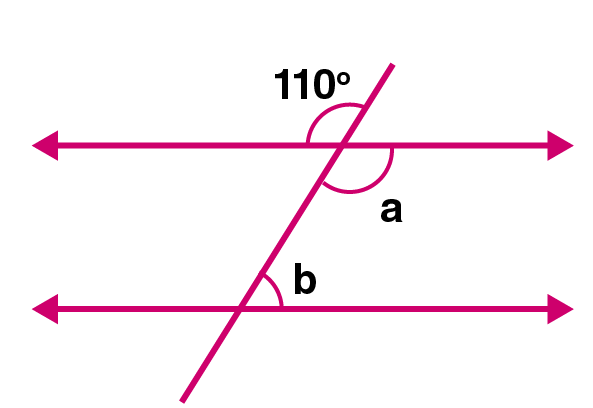(iv)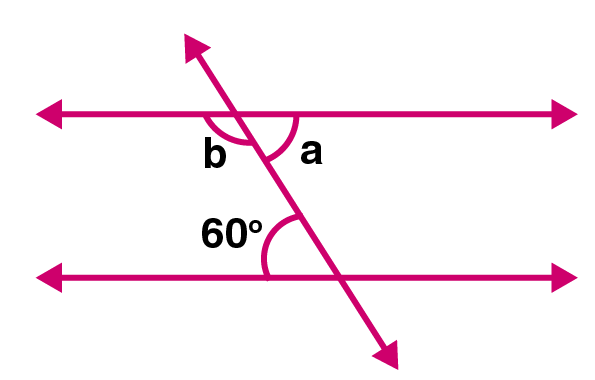(v)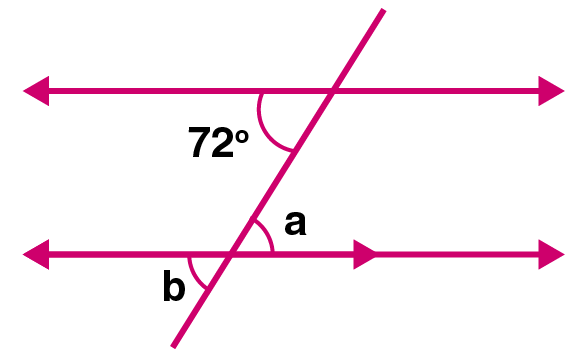Solution:

(i) a + 1400 = 1800 [Linear pair]

a = 1800 – 1400

We get,

a = 400

Here, b = a {alternate angles}

Hence, a = b = 400

(ii) Given

l || m and p intersects them

b + 600 = 1800 [Linear pair]

b = 1800 – 600

We get,

b = 1200

and a = 600 {corresponding angles}

Hence, a = 600

b = 1200

(iii) a = 1100 [Vertically opposite angles]

b = 1800 – a [Co-interior angles]

= 1800 – a

= 1800 – 1100

We get,

= 700

(iv) a = 600 [Alternate interior angles]

b = 1800 – a [Co-interior angles]

= 1800 – 600

We get,

= 1200

(v) a = 720 [Alternate interior angles]

b = a [Vertically opposite angles]

Hence, b = 720

3. If ∠1 = 1200, find the measures of: ∠2, ∠3, ∠4, ∠5, ∠6, ∠7 and ∠8. Give reasons.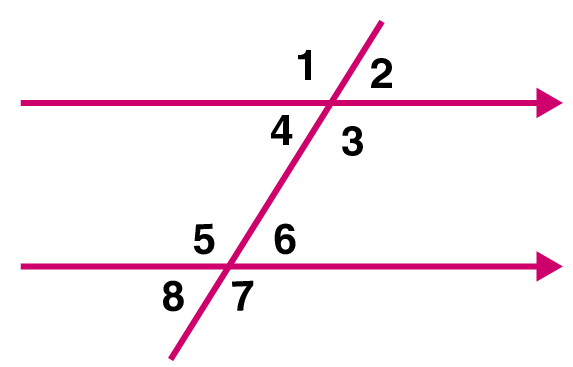Solution:

Given

l || m and p is their transversal and

∠1 = 1200

∠1 + ∠2 = 1800 [Straight line angle]

1200 + ∠2 = 1800

∠2 = 1800 – 1200

We get,

∠2 = 600

Therefore, ∠2 = 600

But ∠1 and ∠3 [Vertically opposite angles]

Hence, ∠3 = ∠1 = 1200

Similarly,

∠4 = ∠2 [Vertically opposite angles]

∠4 = 600

∠5 = ∠1 [Corresponding angles]

Hence, ∠5 = 1200

Similarly,

∠6 = ∠2 [Corresponding angles]

∠6 = 600

∠7 = ∠5 [Vertically opposite angles]

Hence, ∠7 = 1200

and ∠8 = ∠6 [Vertically opposite angles]

Hence, ∠8 = 600

Therefore, the measures of angles are,

∠2 = 600

∠3 = 1200

∠4 = 600

∠5 = 1200

∠6 = 600

∠7 = 1200 and

∠8 = 600

4. In the figure given below, find the measure of the angles denoted by x, y, z, p, q and r.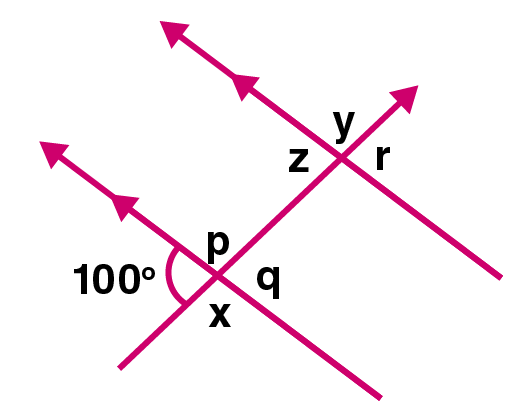Solution:

x = 1800 – 1000 (Linear pair of angles)

x = 800

y = x (Alternate exterior angles)

y = 800

z = 1000 (Corresponding angles)

p = x (Vertically opposite angles)

p = 800

q = 1000 (Vertically opposite angles)

r = q (Corresponding angles)

r = 1000

Therefore, the measures of angles are,

x = y = p = 800

q = r = z = 1000

5. Using the given figure, fill in the blanks.

∠x = …………;

∠z = …………;

∠p = …………;

∠q = …………;

∠r = ………….;

∠s = ………….;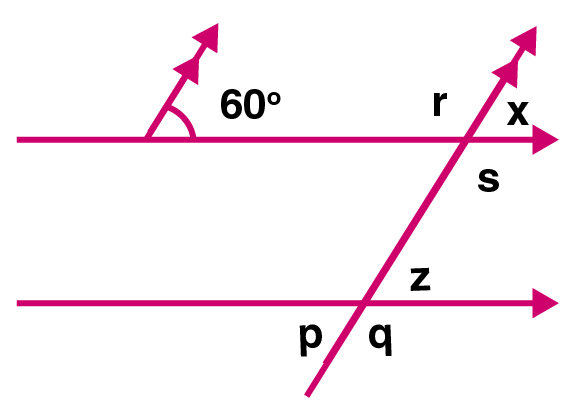Solutions:

∠x = 600 (Corresponding angles)

z = x (Corresponding angles)

= 600

p = z (Vertically opposite angles)

= 600

q = 1800 – p (Linear pair of angles)

= 1800 – 600

We get,

= 1200

r = 1800 – x (Linear pair of angles)

= 1800 – 600

We get,

= 1200

s = r (Vertically opposite angles)

s = r = 1200

6. In the given figure, find the angles shown by x, y, z and w. Give reasons.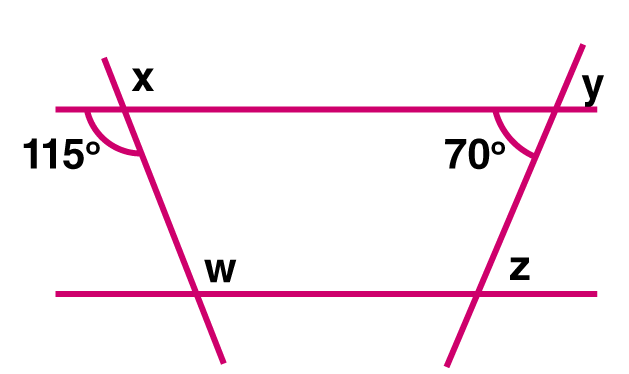Solution:

x = 1150 (By vertically opposite angles)

y = 700 (By vertically opposite angles)

z = 700 (By alternate interior angles)

w = 1150 (By alternate interior angles)

7. Find a, b, c and d in the figure given below: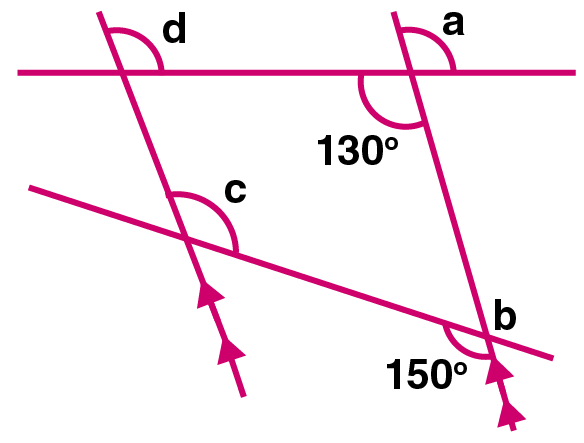Solution:

a = 1300 (By vertically opposite angles)

b = 1500 (By vertically opposite angles)

c = 1500 (By alternate interior angles)

d = 1300 (By alternate interior angles)

8. Find x, y and z in the figure given below: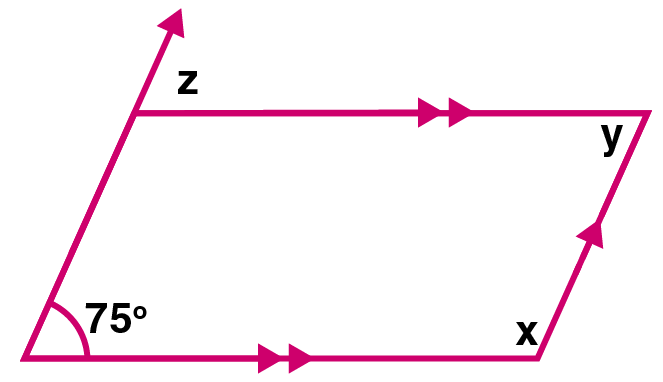Solution:

x = 1800 – 750 (Co-interior angles)

We get,

x = 1050

y = 1800 – x (Co-interior angles)

y = 1800 – 1050

We get,

y = 750

z = 750 (Corresponding angles)

Therefore, the angles are,

x = 1050, y = 750 and z = 750